Home

# THE ROBUSTNESS OF EQUILIBRIA ON CONVEX SOLIDS

## Abstract

We examine the minimal magnitude of perturbations necessary to change the number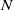$N$ of static equilibrium points of a convex solid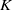$K$ . We call the normalized volume of the minimally necessary truncation robustness and we seek shapes with maximal robustness for fixed values of$N$ . While the upward robustness (referring to the increase of$N$ ) of smooth, homogeneous convex solids is known to be zero, little is known about their downward robustness. The difficulty of the latter problem is related to the coupling (via integrals) between the geometry of the hull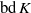$\mathrm{bd} \hspace{0.167em} K$ and the location of the center of gravity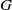$G$ . Here we first investigate two simpler, decoupled problems by examining truncations of$\mathrm{bd} \hspace{0.167em} K$ with$G$ fixed, and displacements of$G$ with$\mathrm{bd} \hspace{0.167em} K$ fixed, leading to the concept of external and internal robustness, respectively. In dimension 2, we find that for any fixed number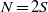$N= 2S$ , the convex solids with both maximal external and maximal internal robustness are regular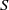$S$ -gons. Based on this result we conjecture that regular polygons have maximal downward robustness also in the original, coupled problem. We also show that in the decoupled problems, three-dimensional regular polyhedra have maximal internal robustness, however, only under additional constraints. Finally, we prove results for the full problem in the case of three-dimensional solids. These results appear to explain why monostatic pebbles (with either one stable or one unstable point of equilibrium) are found so rarely in nature.

## References

Hide All
MathJax
MathJax is a JavaScript display engine for mathematics. For more information see http://www.mathjax.org.

# THE ROBUSTNESS OF EQUILIBRIA ON CONVEX SOLIDS

## Metrics

### Full text viewsFull text views reflects the number of PDF downloads, PDFs sent to Google Drive, Dropbox and Kindle and HTML full text views.

Total number of HTML views: 0
Total number of PDF views: 0 *Loading metrics...

### Abstract viewsAbstract views reflect the number of visits to the article landing page.

Total abstract views: 0 *Loading metrics...

* Views captured on Cambridge Core between <date>. This data will be updated every 24 hours.

Usage data cannot currently be displayed